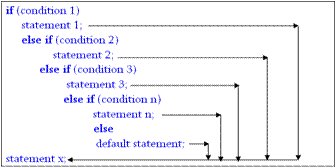• When we have multiple options available or we need to take multiple decisions based on available condition, we can use another form of if statement called else…if ladder.
• In else…if ladder each else is associated with another if statement.
• Evaluation of condition starts from top to down.
• If condition becomes true then the associated block with if statement is executed and rest of conditions are skipped.
• If the condition becomes false then it will check for next condition in a sequential manner.
• It repeats until all conditions are cheeked or a true condition is found.
• If all condition available in else…if ladder evaluated to false then default else block will be executed.
• It has the following syntax:

Syntax:

``````if (condition 1)
{
// block 1
}
elseif (codition 2)
{
// block 2
}
elseif(codition 3)
{
// block 3
}
else
{
// default block
}``````• else…if ladder provides multi way decision making when any one alternative is to be select among the available option.
• The following block demonstrates the use of else if ladder.
``````<?php
\$x=10, \$y=20, \$ch = 3, \$z = 0 ;

if (\$ch == 1) {
\$z = \$x + \$y;
}
elseif (\$ch == 2){
\$z = \$x - \$y;
}
elseif(\$ch == 3){
\$z = \$x * \$y;
}
elseif(\$ch == 4){
\$z = \$x/\$y ;
}
else{
echo "Invalid choice! Please try again!" ;
}
echo "Answer is \$z" ;
?>
``````